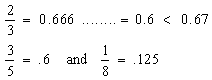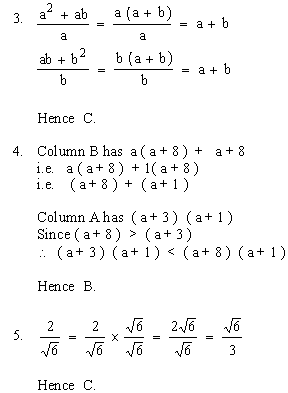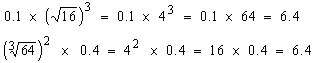Please Support the Monkey! Tell All your Friends and Teachers
 PRACTICAL TEST 2 Section IV : Quantitative SectionANSWER EXPLANATIONS Test Paper IIOne, out of the 3 terms of column A, is less than that of B, the other two equal. Hence B. Number of minutes in 7 days = 7 (days) ´ 24 (hrs) ´ 60 minutes = 7 ´ 24 ´ 60 Number of hours in 60 weeks = 60 (weeks) ´ 7 (days) ´ 24 hours = 60 ´ 7 ´ 24 Hence C.Area of square = ( 1.8 )2 = 3.24 Area of circle = p r2 = p ( 1 )2 = p = 3.1415......... Hence A. Side AB is opposite Ð C = 500 Side AC is oppowite Ð B = 800 and the side opposite the greater angle is the greater side. \ AC > AB Hence B. Ð A = 180 - ( Ð B + Ð C ) = 180 - 130 = 50 Hence A. ( i.e. column A or Ð B ). Ð B = 800 < 900 \ AC2 < AB2 + BC2 Hence A.Hence C. Test 2 Section 1 : Verbal Section Section 2 : Quantitative Section Section 3 : Analytical Section Section 4 : Quantitative Section Section 5 : Verbal Section Section 6 : Analytical Section Answer Key To Test 2 Answer Explanation To Test 2 Section 1 : Verbal Section Section 2 : Quantitative Section Section 3 : Analytical Section Section 4 : Quantitative Section Section 5 : Verbal Section Section 6 : Analytical Section